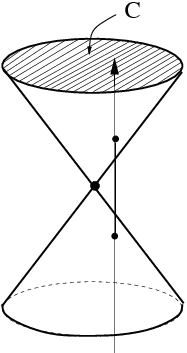# CSE 599: Recent Developments in Approximation Algorithms

In this course we study several of recent papers in the field of approximation algorithms.
We focus on many of the underlying techniques including randomized rounding,
spectral methods, random walks and real stable polynomials.

• Instructor: Shayan Oveis Gharan
• Office Hours: By appointment, email me at shayan at cs dot washington dot edu.
• Lectures: Monday - Wednesday 4:00 - 5:20 at EEB 031

### Related Materials

Lecture Topic Notes Bonus Materials
Lecture 1 (03/30/15) Background, Matrix Tree Theorem tex, pdf
Lecture 2 (04/01/15) Negative Correlation and Burton and Pemantle Theorem
Lecture 3 (04/06/15) Characteristic Polynomials and proof of Burton,Pemantle Theorem tex, pdf
Lecture 4 (04/08/15) Electrical Flows and Effective Resistance tex, pdf
Lecture 5 (04/13/15) Effective Resistance and Random Walks
Lecture 6 (04/15/15) An O(logn/loglogn) Approximation Algorithm for Asymmetric TSP tex, pdf
Lecture 7 (04/20/15)
Lecture 8 (04/22/15)
Lecture 9 (04/27/15) The Pipage Rounding Method tex, pdf
Lecture 10 (04/29/15) Hyperbolic and Real Stable Polynomials tex, pdf
Lecture 11 (05/04/15) Interlacing and Strongly Rayleigh distributions
Lecture 12 (05/06/15) A Randomized Rounding Algorithm for STSP tex, pdf
Lecture 13 (05/11/15) A Construction of Linear size Thin Forests tex, pdf
Lecture 14 (05/13/15) Spectrally Thin Subsets using Interlacing Polynomials tex, pdf
Lecture 15 (05/18/15) Kadison-Singer Conjecture and its Extensions tex, pdf
Lecture 16 (05/20/15) Proof of the Kadison Singer Conjecture
Lecture 17 (06/01/15) Cheeger's Inequality and the Sparsest Cut Problem tex, pdf
Lecture 18 (06/03/15) Existence of Spectrally Thin Trees tex, pdf
Lecture 19 (05/08/15) The Dual of Effective Resistance CP

### Tentative Agenda

• Random Spanning Trees and Determinantal Measures
• Electrical Flows and Effective Resistance
• Rounding by Sampling Method and O(log n/loglog n) approximation algorithm for ATSP
• Randomzied Rounding by Pipage Rounding Method
• Randomized Rounding Algorithm for the Steiner Tree Problem
• Real Stable and Hyperbolic Polynomials
• Strongly Rayleigh Distributions and their properties
• Approximation Algorithms for Symmetric TSP
• Interlacing Polynomials and the Bourgain-Tzafriri Theorem
• Kadison Singer Problem and the Thin Basis Problem
• Thin Edge Covers and Improved Approximation Algorithms for Asymmetric TSP
• Spectral Graph Theory and Cheeger Inequality
• Subspace Enumeration Method and Subexponential time algorithms for Unique Games Problem
• Higher Order Cheeger Inequality
• Sum of Squares and Approximation Algorithms for Low Threshold Rank Graphs